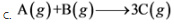# At constant pressure, which of these systems do work on the surroundings? Check all that apply

At constant pressure, which of these systems do work on the surroundings? Check all that apply.

1. 2A(g)+3B(g) --> 4C(g)
2. A(s)+B(g) --> 2C(g)
3. A(g)+B(g) --> 3C(g)
4. A(s)+2B(g) --> C(g)

Concepts and reason
The concept used to solve this problem is thermodynamics and work done during expansion.
If the work done is done on the surroundings by the system, then the work done will be negative. Hence, for the work done to be negative, it is necessary that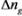is positive for the system under evaluation.

Fundamentals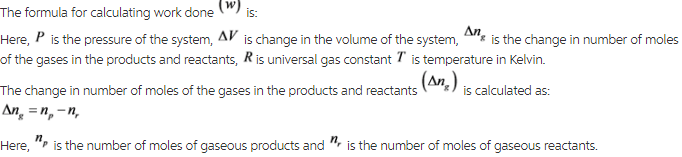Consider the following reaction a:Therefore, work is not done by the system (reaction a) on the surroundings.
Consider the following reaction d: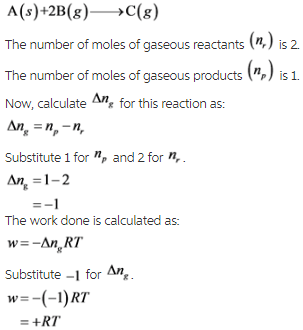Therefore, work is not done by the system (reaction d) on the surroundings.
When the work done is negative, it indicates that the work is done by the system on the surroundings.

Consider the following reaction b: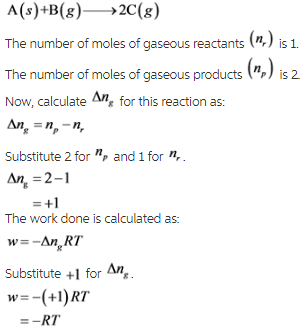Therefore, work is done by the system on the surroundings.
The following system does work on the surrounding: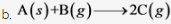When the work done is negative, it indicates that the work is done by the system on the surroundings. Also, it is assumed in the reaction of solid and gas, no fluidization occurs, otherwise its volume expansion must be taken into account and no values/information is given in the question itself.

Consider the following reaction c: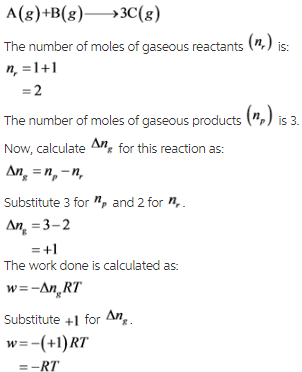Therefore, work is done by the system on the surroundings.
The following system does work on the surrounding: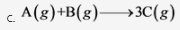When the work done is negative, it indicates that the work is done by the system on the surroundings. Also, it is assumed in the reaction of solid and gas, no fluidization occurs, otherwise its volume expansion must be taken into account and no values/information is given in the question itself.
The following system does work on the surrounding: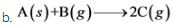The following system does work on the surrounding: